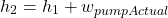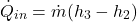# Rankine Cycle – Principles and Calculations

#### Engineering

The Rankine cycle is a thermodynamic cycle that allows uses steam as the working fluid inside of a cycle. This contrasts with most thermodynamic cycles that use air or some sort of fuel. It can also have reheat or even regenerative components to increase efficiency. In this article, you will learn the principles of the Rankine cycle, calculation of values, and variations of the cycle.

## Principles of the Rankine Cycle

The Rankine cycle consists of four main components: pump, boiler, turbine, and condenser. The process starts with the pump bringing in water into the system and increasing pressure via an Isentropic compression process. Next, the water is heated in a boiler, traditionally via fossil fuel combustion and an isobaric process. After the water is turned into steam and sent to the next stage. The steam then runs through a turbine and undergoes an isentropic expansion. Finally, the vapor is put into a condenser and made back into liquid water in an isobaric process.

## Calculations of the Rankine Cycle

An in-depth look at the Rankine cycle involves finding the enthalpies at each stage of the cycle, the heat addition and loss, and work entering and exiting the system. Understanding cycle efficiencies of the cycle allows improvements to occur through changes of pressure or temperature or different parts.

During the Rankine cycle calculations, there are a few variables to track to fully represent the cycle. Since the working fluid in this cycle is water, steam tables will be used to find different variables at certain points. This cycle also has an ideal and an actual version. The ideal version has the theoretical efficiency as 100% and the actual is usually between 30-80% depending on how the cycle is designed. The goal of the calculations is to find the enthalpies at each stage to analyze how efficient the cycle will be.

### Stage 1 ➔ 2 (Pump)

Quality measures the proportion of liquid to steam. If the user assumes the quality is zero (x1) and knows the pressure leaving the condenser (P1), enthalpy can be determined at state 2.

#### Ideal

First, obtain the enthalpy (h1), entropy (s1), and specific volume (ν1) which is equal to the (hf, sf, νf) values at the given pressure (P1). Next, find the work the pump did by using pressure (P2).Next, use the pump work to find the enthalpy(h2) at state 2.#### Actual

In the actual cycle, the variable of pump efficiency(ηp) influences the amount of work placed into the cycle. Next the enthalpy(h1), entropy(s1), and specific volume(νf) are still equal to (hf, sf, νf) at the given pressure(P1). These variables determine the actual work of the pump.Next, the pump work determines the enthalpy(h2).### Stage 2 ➔ 3 (Boiler)

The pressure at stage 3 equals the pressure at stage 2 (P3 = P2) and the given temperature after the boiler(T3).

Use the pressure and temperature to find the enthalpy (h3) and entropy (s3) at stage three of the cycle from the pressure and temperature. This is done via steam tables and taking the quality of the steam to be (x3 = 1), which is a superheated vapor.

### Stage 3 ➔ 4 (Turbine)

Given the temperature going into the turbine(T3) or the pressure into the turbine(P3), and then using those with the steam tables to find the enthalpy at state 4.

#### Ideal –

The entropy(s4) is equal to (s3). Use this relationship to find the quality of the vapor at stage four.Next, use the found quality to find the enthalpy(h4).#### Actual

Since its isentropic, the entropy(s4) is equal to (s3). Use the entropy to find the quality of the vapor after the turbine.Then using the quality to find the enthalpy (h4).### Stage 4 ➔ 1 (Condenser)

The pressure at stage 4 is equal to that of stage one(P4 = P1).

#### Ideal –

Lastly finding the work across the turbine as the stage 1 values of the Rankine cycle have been previously found.#### Actual –

Finally, take the efficiency of the turbine (ηt) to determine actual turbine work.### Other Calculations of the Rankine Cycle

#### Ideal

There are different equations that show the transfer of heat to and from the system and the work performed by various components. The mass transfer rate() helps determine this information.#### Actual## Variations of the Rankine Cycle

### Reheat

Reheating in the Rankine cycle happens after the first turbine. The steam runs back through the boiler, reheats, and then goes through a second turbine. This process stops the vapor from condensing inside the turbine and prevents damage to the blades. A reheat Rankine cycle also improves the efficiency of the cycle all around because it adds more ability to capture the remaining energy leftover from the first turbine.

### Regeneration

Regeneration in the Rankine cycle is the process of mixing liquids at different stages of the cycle to induce direct contact heating. This raises the average temperature across the cycle by using fractional steam to make the initial water hotter. This causes less fuel to be used because excess heat normally lost to the environment preheats the fluid.